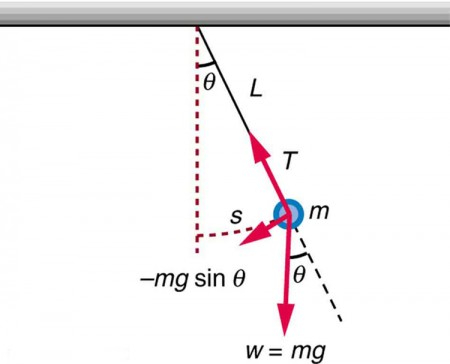# What are the formulas related to pendulum?

Pendulum is a combination of weight hung from a fixed point. The arrangement is in such a way that it allows the device to swing to and fro freely.

Formula's related to the pendulumTime period

This equation helps us to calculate how long the pendulum takes to swing to and fro. It is denoted by T.

$T\ =\ 2\pi \sqrt{\frac{L}{g}}$

Where, T = Time period in seconds,

L = Length of the rod or wire.

g = gravitational acceleration

Frequency

This calculates the number of times a pendulum swings to and fro in a second. It is measured in hertz.

$f\ =\ \frac{1}{T}$ Where f = Frequency in hertz,

T = Time period in seconds.

Updated on: 10-Oct-2022

36 Views